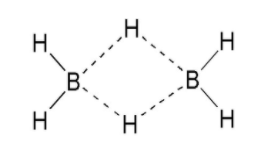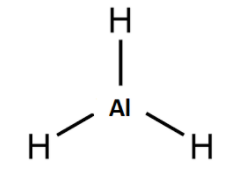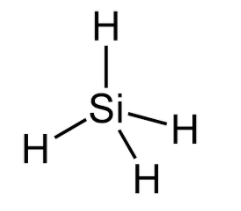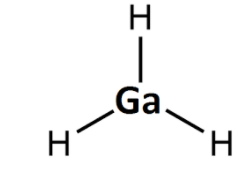QuestionAnswers

# The hydride that is electron deficient is:(A) ${{B}_{2}}{{H}_{6}}$(B) $Al{{H}_{3}}$(C) $Si{{H}_{4}}$(D) $Ga{{H}_{3}}$Verified
91.8k+ views
Hint: To solve this question we have to determine the octet or duplet of each atom in the molecule. If the octet and duplet of each of the atoms in the molecule is complete then it is not electron deficient.

Complete step by step solution:
To determine whether the molecule is electron deficient or not we should determine that the atoms in the molecule have their complete octet i.e. eight electrons in the outermost shell or duplet i.e. 2 electrons in the outermost shell. Duplet is only applicable in some of the atoms.
${{B}_{2}}{{H}_{6}}$Boron has 3 electrons in the valence shell and it requires 5 electrons to complete its octet.
From the structure we can see that diborane has a 3c-2e bond, hence it is electron deficient.
$Al{{H}_{3}}$Aluminium has 3 electrons in the valence shell and it requires 5 electrons to complete its octet , if it forms a bond with 3 hydrogen it still requires more electrons to complete its octet. Hence it is electron deficient.
$Si{{H}_{4}}$Silicon has 4 electrons in its valence shells and requires 4 electrons to complete its octet and here it forms a bond with 4 hydrogen atoms hence $Si{{H}_{4}}$ is an electron precise molecule.

Hence the correct answer is option (C) i.e. $Si{{H}_{4}}$ is an electron precise molecule not electron deficient.

$Ga{{H}_{3}}$Gallium has 3 valence electrons and it requires 5 electrons to complete its octet, if it forms a bond with 3 hydrogen it still requires more electrons to complete its octet. Hence $Ga{{H}_{3}}$ is electron deficient.

Note: In order to determine a complete octet we should determine its chemical structure and to draw the chemical structure we have to use the VSEPR theory or the hybridization concept. We can determine the hybridization by adding the number of lone pairs and the number of sigma bonds.